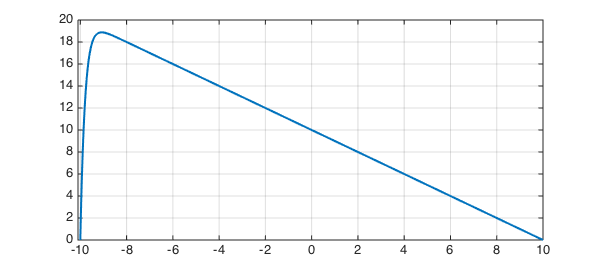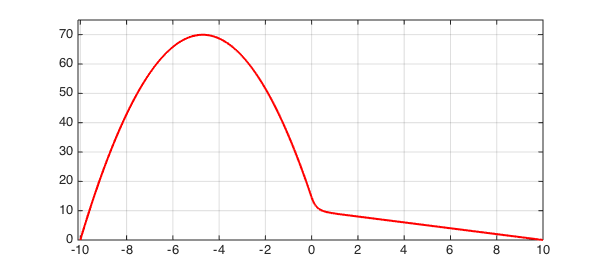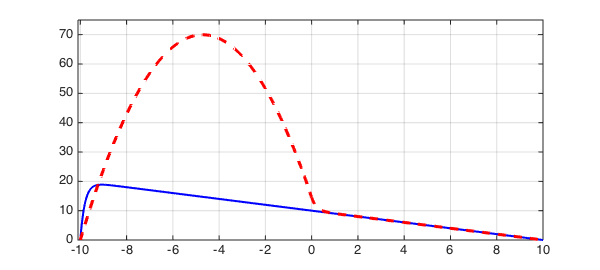The solution to the advection-diffusion problem

$$0.2u'' + u' = -1, ~~ u(-10) = u(10) = 1$$

has a boundary layer at the left:

LW = 'linewidth'; lw = 2; FS = 'fontsize'; fs = 8;
N = chebop(-10,10);
N.op = @(u) 0.2*diff(u,2) + diff(u);
N.bc = 'dirichlet';
u = N\-1;
plot(u,LW,lw), grid on
axis([-10.1 10 0 20])Suppose the advection is only turned on on the right half of the domain?

figure
N.op = @(x,u) 0.2*diff(u,2) + (x>=0).*diff(u);
N.bc = 'dirichlet';
v = N\-1;
plot(v,'r',LW,lw), grid on
axis([-10.1 10 0 75])For fun we can plot both solutions on the same axis.

plot(u,'b',v,'--r',LW,lw), grid on
axis([-10.1 10 0 75])